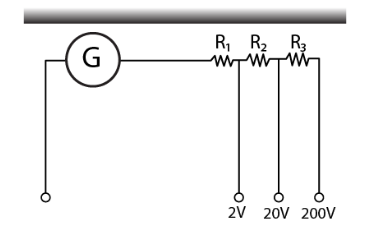Deepak Scored 45->99%ile with Bounce Back Crack Course. You can do it too!

# A multirange voltmeter can be constructed by using

Question:

A multirange voltmeter can be constructed by using a galvanometer circuit as shown in the figure. We want to construct a voltmeter that can measure 2V, 20V, and 200V using galvanometer of resistance 10Ω and that produces maximum deflection for a current of 1 mA. Find R1, R2, and R3 that have to be used.Solution:

iG(G+R1) = 2 for 2V range

iG(G+R1+R2) = 20 for 20V range

iG(G+R1+R2+R3) = 200 for 200V range

Solving the above, we get

R1 = 1990 Ω

R2 = 18kΩ

R3 = 180 kΩ